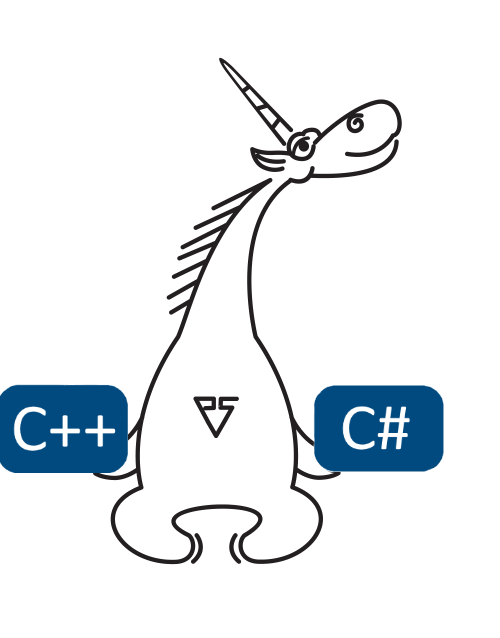﻿ Type Conversion in C++ and C# Arithmetic Expressions# Type Conversion in C++ and C# Arithmetic Expressions

In arithmetic expressions, the types of operands can be converted to a common type. Such conversions are described in the language standard, and in C# they are much simpler than in C++. However, I'm not sure that many programmers know all the details.

Perhaps you had situations when the type of an arithmetic expression turned out to be something different from what you had expected. How well do you know the language standard? Test yourself by replacing auto and var with appropriate types in the expressions below and evaluating these expressions:

C++ (we're assuming that an LP64 data model is used):

``````void Test()
{
unsigned char c1 = std::numeric_limits<unsigned char>::max();
unsigned char c2 = std::numeric_limits<unsigned char>::max();
int i1 = std::numeric_limits<int>::max();
int i2 = std::numeric_limits<int>::max();
unsigned int u1 = std::numeric_limits<unsigned int>::max();

auto x = c1 + c2;
auto y = i1 + i2;
auto z = i1 + u1;
}``````

C#:

``````void Test()
{
byte b1 = byte.MaxValue;
byte b2 = byte.MaxValue;
int i1 = int.MaxValue;
int i2 = int.MaxValue;
uint u1 = uint.MaxValue;

var x = b1 + b2;
var y = i1 + i2;
var z = i1 + u1;
}``````

The answer is below the pictureC++ (LP64):

``````    int x = c1 + c2;          // = 510
int y = i1 + i2;          // = -2
unsigned int z = i1 + u1; // = 2147483646``````

C#:

``````    int x = b1 + b2;          // = 510
int y = i1 + i2;          // = -2
long z = i1 + u1;         // = 6442450942``````

Here is what follows from this test - or rather the C++ and C# standards:

1. Evaluation of x. In an arithmetic expression, all the variables whose values can be represented with type int will be converted to this type, so when adding two variables of type char, unsigned char, short int, or unsigned short int in C++, or variables of type byte, sbyte, short, or ushort in C#, the resulting value will be of type int and no overflow will occur. In our examples, the x variable will take the value 510.

2. Evaluation of y. If both arguments are of type int, no further type promotion will take place and an overflow is possible. In C++, an overflow leads to undefined behavior. In C#, the application will continue running by default. You can use the checked keyword or /checked compiler switch to change its behavior so that it raises an OverflowException in the case of an overflow. In our test, the y variable will take the value -2 both in C++ and C#. However, remember that in C++ we'll be dealing with undefined behavior, which may manifest itself in any way - for example writing the number 100500 to y or ending up with a stack overflow.

3. Evaluation of z. The situation when one of the arguments is of type int and the other is of type unsigned int in C++ or uint in C# is handled differently by each standard! In C++, both arguments will be converted to type unsigned int. By the way, if an overflow occurs, it wouldn't be an undefined behavior. In C#, both arguments will be converted to type long and no overflow will be ever possible. It is the reason why we got different values for the z variable in our programs in different languages.

Now let's see what errors can be found in code written without taking type-conversion rules into account.

C++ example:

``````typedef unsigned int    Ipp32u;
typedef signed int      Ipp32s;

Ipp32u m_iCurrMBIndex;

VC1EncoderMBInfo* VC1EncoderMBs::GetPevMBInfo(Ipp32s x, Ipp32s y)
{
Ipp32s row = (y > 0) ? m_iPrevRowIndex : m_iCurrRowIndex;
return ((m_iCurrMBIndex - x < 0 || row < 0)
? 0 : &m_MBInfo[row][m_iCurrMBIndex - x]);
}``````

This code fragment is taken from IPP Samples project. When comparing an expression result with zero, one should keep in mind that int may be cast to unsigned int, and long to unsigned long. In our case, the result of the m_iCurrMBIndex - x expression will be of type unsigned int, so it is always nonnegative - PVS-Studio will warn you about this issue: V547 Expression 'm_iCurrMBIndex - x < 0' is always false. Unsigned type value is never < 0.

C# example:

``````public int Next(int minValue, int maxValue)
{
long num = maxValue - minValue;
if (num <= 0x7fffffffL)
{
return (((int)(this.Sample() * num)) + minValue);
}
return (((int)((long)(this.GetSampleForLargeRange() * num)))
+ minValue);
}``````

This sample is taken from SpaceEngineers project. In C#, you should always keep in mind that when adding two variables of type int, their type will never be promoted to long, unlike the situation when you add a variable of type int and a variable of type uint. Therefore, what will be written to the num variable is an int value, which always meets the num <= 0x7fffffffL condition. PVS-Studio knows about this issue and generates the message V3022 Expression 'num <= 0x7fffffffL' is always true.

It's great when you know the standard and know how to avoid errors like those discussed above, but in real life remembering all the intricacies of language is difficult - and totally impossible in the case of C++. And here's where static analyzers like PVS-Studio may be of help.

## References##### Use PVS-Studio to search for bugs in C, C++, C# and Java

We offer you to check your project code with PVS-Studio. Just one bug found in the project will show you the benefits of the static code analysis methodology better than a dozen of the articles.

412
14 132

### Do you make errors in the code?